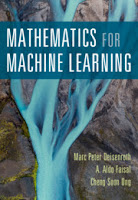## Monday, October 14, 2019

### Suggested Books Today | Books - Helge Scherlund's eLearning News

Check out these books below by Cambridge University Press.Photo:
Machine Learning Refined - Foundations, Algorithms, and ApplicationsMachine Learning RefinedFoundations, Algorithms, and Applications
With its intuitive yet rigorous approach to machine learning, this text provides students with the fundamental knowledge and practical tools needed to conduct research and build data-driven products. The authors prioritize geometric intuition and algorithmic thinking, and include detail on all the essential mathematical prerequisites, to offer a fresh and accessible way to learn...

A suite of online resources including sample code, data sets, interactive lecture slides, and a solutions manual are provided online, making this an ideal text both for graduate courses on machine learning and for individual reference and self-study.

A suite of online resources including sample code, data sets, interactive lecture slides, and a solutions manual are provided online, making this an ideal text both for graduate courses on machine learning and for individual reference and self-study.
• Encourages geometric intuition and algorithmic thinking to provide an intuitive understanding of key concepts and an interactive way of learning
• Features coding exercises for Python to help put knowledge into practice
• Emphasizes practical applications, with real-world examples, to give students the confidence to conduct research, build products, and solve problems
• Completely self-contained, with appendices covering the essential mathematical prerequisites
Publication planned for: January 2020

Understanding Machine Learning - From Theory to AlgorithmsUnderstanding Machine LearningFrom Theory to Algorithms
Machine learning is one of the fastest growing areas of computer science, with far-reaching applications. The aim of this textbook is to introduce machine learning, and the algorithmic paradigms it offers, in a principled way. The book provides a theoretical account of the fundamentals underlying machine learning and the mathematical derivations that transform these principles into practical algorithms...

Designed for advanced undergraduates or beginning graduates, the text makes the fundamentals and algorithms of machine learning accessible to students and non-expert readers in statistics, computer science, mathematics and engineering.
• Provides a principled development of the most important machine learning tools
• Describes a wide range of state-of-the-art algorithms
• Promotes understanding of when machine learning is relevant, what the prerequisites for a successful application of ML algorithms are, and which algorithms to use for any given task
Date Published: July 2014

Mathematics for Machine LearningMathematics for Machine Learning
The fundamental mathematical tools needed to understand machine learning include linear algebra, analytic geometry, matrix decompositions, vector calculus, optimization, probability and statistics. These topics are traditionally taught in disparate courses, making it hard for data science or computer science students, or professionals, to efficiently learn the mathematics...

For those learning the mathematics for the first time, the methods help build intuition and practical experience with applying mathematical concepts. Every chapter includes worked examples and exercises to test understanding. Programming tutorials are offered on the book's web site.
• A one-stop presentation of all the mathematical background needed for machine learning
• Worked examples make it easier to understand the theory and build both practical experience and intuition
• Explains central machine learning methods: linear regression, principal component analysis, Gaussian mixture models and support vector machines
Publication planned for: January 2020

Linear Algebra and Learning from DataLinear Algebra and Learning from Data
Linear algebra and the foundations of deep learning, together at last! From Professor Gilbert Strang, acclaimed author of Introduction to Linear Algebra, comes Linear Algebra and Learning from Data, the first textbook that teaches linear algebra together with deep learning and neural nets...

• The first textbook designed to teach linear algebra as a tool for deep learning
• From Professor Gilbert Strang, acclaimed author of Introduction to Linear Algebra
• Includes the necessary background from statistics and optimization
• Explains stochastic gradient descent, the key algorithim of deep learning, in detail
Date Published: January 2019